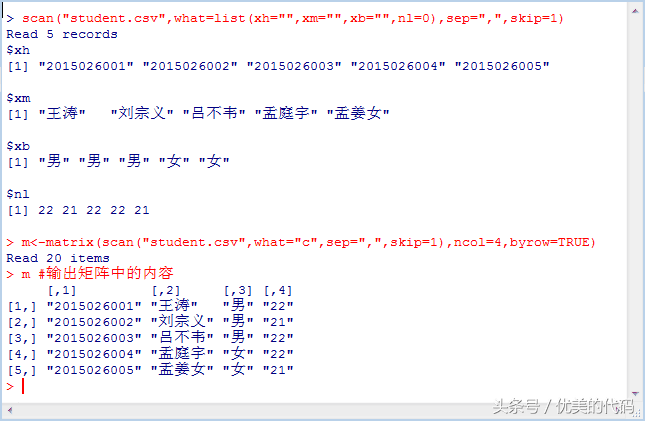# scan读取数据读取文本文件所用的函数是(读取文本文件所用的函数是)

R语言中的scan函数除了可以从键盘获取数据外，还可以从文件中读取数据，下面将介绍如何从文本文件以及CSV文件中读取数据的方法。从CSV文件中读取数据CSV文件，默认是以逗号进行分隔的数值文件。使用scan()函数读取csv格式的文件，与读取文本型数据没有多大区别，不过要指定逗号作为分隔符。将student.txt文件中的数据键入到Excel文件后，另存为student.csv，并将其放到R的工作

R语言中的scan函数除了可以从键盘获取数据外，还可以从文件中读取数据，下面将介绍如何从文本文件以及CSV文件中读取数据的方法。从CSV文件中读取数据CSV文件，默认是以逗号进行分隔的数值文件。使用scan()函数读取csv格式的文件，与读取文本型数据没有多大区别，不过要指定逗号作为分隔符。将student.txt文件中的数据键入到Excel文件后，另存为student.csv，并将其放到R的工作目录中。欢迎关注本号，本号近期将持续更新有关R语言方面的文章，内容将由浅入深，从基本语法到数据分析，逐渐步入R语言的世界。如有疑问或建议请给我留言。

R语言中的scan函数除了可以从键盘获取数据外，还可以从文件中读取数据，下面将介绍如何从文本文件以及CSV文件中读取数据的方法。

# 从文本文件中读取数据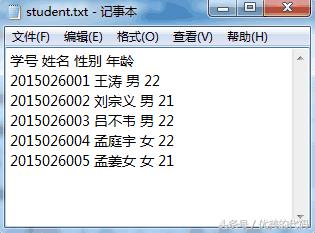（1）scan("student.txt", what="c") #以字符串的格式读取数据

（2）scan("student.txt", what="c", nlines=3) #读取3行

（3）scan("student.txt", what="c", skip=1) #忽略第1行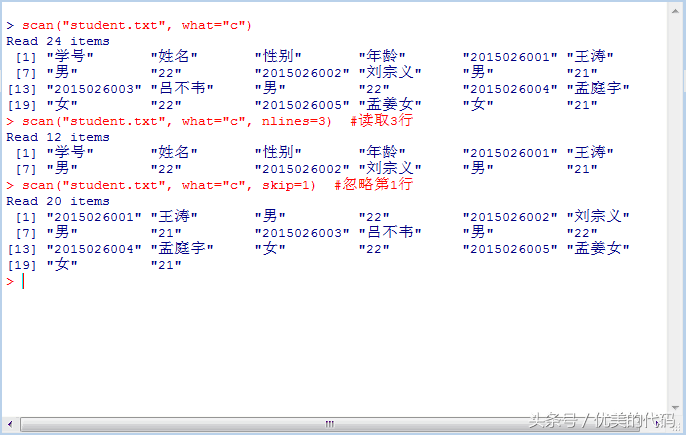scan函数读取文本文件中的数据

（4）scan("student.txt", what = list(studentNo="", studentName="", studentSex="", studentAge=0), skip=1) #以列表的形式读取数据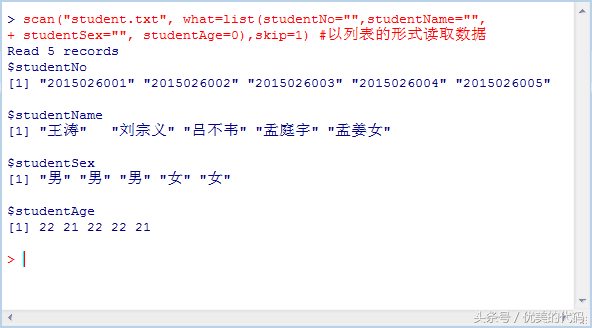scan函数以列表形式读取文件中的数据

（5）lst <- scan("student.txt", what = list(xh="", xm="", xb="", nl=0), skip=1) #读取数据并保存到变量中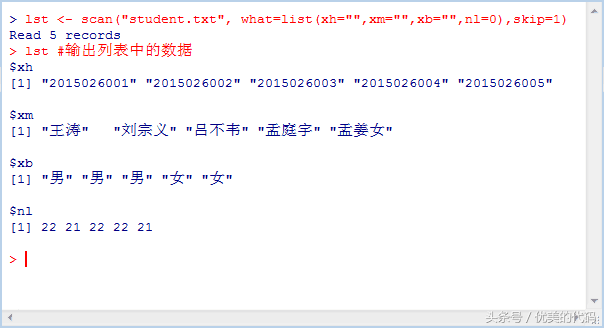scan函数读取文件数据到变量中

# 从CSV文件中读取数据

CSV文件，默认是以逗号进行分隔的数值文件。将Excel文件另存CSV格式的文件后，可以使用R语言的scan()函数读取其数据。

（1）scan("student.csv") #scan()函数默认以double格式存储数据，而该文件中包含不能转换的数据而报错

（2）scan("student.csv", what="c") #由于未指定分隔符，scan()函数将每一行作为一个数据域

（3）scan("student.csv", what="c", sep=",") #指定逗号作为分隔符后，可以正确读取数据了

（4）scan("student.csv", what="c", sep=",", skip = 1) #忽略第一行的标题行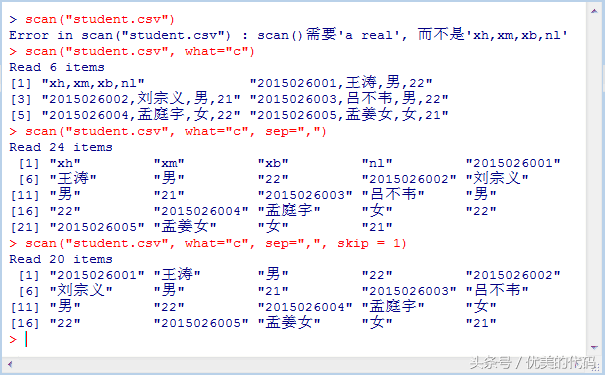scan函数从csv文件中读取数据时的情况

（5）scan("student.csv", what = list(xh="", xm="", xb="", nl=0), sep=",", skip=1) #以列表的形式读取数据

（6）m <- matrix(scan("student.csv", what="c", sep=",", skip=1), ncol=4, byrow=TRUE) #读取数据并创建矩阵